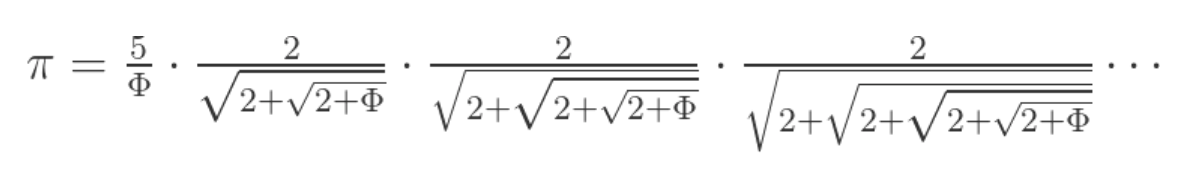# What are some interesting relationships between pi and phi? [closed]

Phi is the golden mean solution to the 1/x=1+x and pi the transcendental number relating the radius of the circle to its area.

A side note: while there are really interesting series converging to pi, I thing it's impossible to give a coordinate free proof it's equivalence to the ratio between the area and radius if a circle.

Is there any way to use the golden ratio to define pi, that could then possibly lead to a coordinate less relationship between geometric pi and pi as a series?

Q: Is there any way to use the golden ratio $$\Phi$$ to define $$\pi$$ ?